New Features in Maple 16: 3-D Interpolation and SmoothingCreating 3-D plots from discrete data has never been easier using the new smoothing and interpolation techniques in Maple 16. The smoothing algorithm allows you to generate a smooth surface that approximates your noisy data. The interpolation method generates a surface which matches your data points exactly, regardless of whether the data points lie on a uniform or non-uniform grid.

Details and Examples

 > with(LinearAlgebra): with(CurveFitting): with(plots): with(Statistics): randomize():

Smoothing

In this example, we are assuming that we have a collection of 2-dimensional independent data for which the 1-dimensional dependent data has some error component or is known to be noisy. The presence of noise implies that whatever surface this data represents does not necessarily pass through the given dependent data points. In this case, a surface is approximated by numerically smoothing the data using the lowess algorithm. For any given point to be plotted a window of close enough, surrounding data points will be used to compute a local, weighted, low order fit.

The new command ScatterPlot3D, from the Statistics package, provides a smoothed plot of the 2-D noisy data.

The independent data does not need to be regularly spaced, and is supplied as an n-by-3 Array or Matrix. Each of the n rows represents an individual point. The columns are the  x-, y-, and z-values.

Here is an example. First, we'll look down upon the projection of the data points onto the x-y plane, and thus visualize the layout and spacing of the 2-D dependent data values.

 > X := Sample(Uniform(-50,50),175):

 > Y := Sample(Uniform(-50,50),175):

 > Zerror := Sample(Normal(0,100),175):

 > Z := Array(1..175,(i)->-(sin(Y[i]/20)*(X[i]-6)^2+(Y[i]-7)^2+Zerror[i])):

 > XYZ := Matrix([[X],[Y],[Z]],datatype=float)^%T;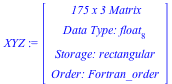> ScatterPlot3D(XYZ, axes=box, orientation=[20,0,0]);> ScatterPlot3D(XYZ, lowess, grid=[25,25], axes=box, orientation=[20,70,0]);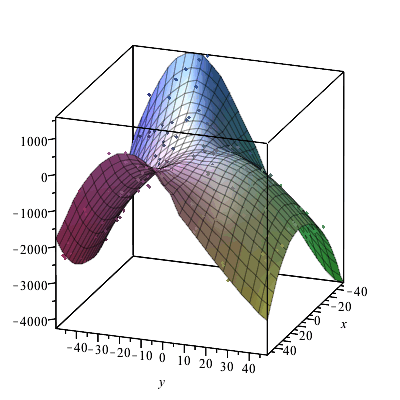Here is another example, which reads in the n-by3 data from a file.

 > M:=ImportMatrix(cat(kernelopts(mapledir),"/data/plotting/irregulardata3D.csv"),                source=csv,datatype=float):

 > Ppt := pointplot3d(M,axes=box,style=patchnogrid,symbolsize=15,color=red,                          labels=[x,y,z]):

 > display(Ppt,orientation=[90,0,0]);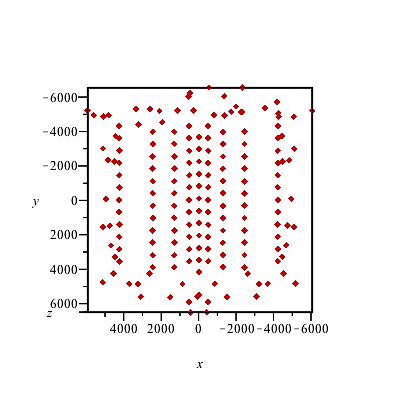The default options for the ScatterPlot3D command are to use an order 2 fitting quadratic form, and to use a window of width 1/3 of the original domain in each of the x- and y-directions.

On the right below is the smoothed surface, while on the left is a slightly transparent view of the same surface overlaid with a point-plot of the original data points.

 > Ploess := ScatterPlot3D( M, lowess ):

 > display(Array([         display([Ppt,Ploess]),         display(Ploess,style=patch,color=RGB(0.0,0.4,0.6))                      ]),view=700..860,axes=box,orientation=[54,78,-15],                         transparency=0.0);Interpolation

In these examples, we are assuming that we have a collection of 2-dimensional independent data for which the 1-dimensional dependent data is accepted as correct. The goal is to produce a surface which must must pass through all of the data points. That is, at every x-y point of the independent data the height of the plotted surface must match the corresponding value of the dependent (z) data. In this case, a surface is computed numerically by interpolating the data.

New in Maple 16, it is now possible to produce an interpolated 3-D surface using both regular and irregularly spaced data.

Uniform data grid

In the case of a uniform 2-dimensional grid of data a surface plot can be generated using the surfdata command.

The data is comprised of a grid of x- and y-points. For the examples of this section, the data points are taken uniformly in each direction. The x-values are taken as as the integers from 1 to 7, and the y-values are taken as the integers from 1 to 9.

View of data points in the x-y plane

Here is a view of the input data points, which in this example is a full grid, uniformly spaced in both the x and y directions.

 > xpts:=<(\$1..7)>: ypts:=<(\$1..9)>:

 > display( seq(plottools:-line([xpts[i],1],[xpts[i],9]), i=1..7),         seq(plottools:-line([1,ypts[j]],[7,ypts[j]]), j=1..9),         pointplot([seq(seq([xpts[i],ypts[j]],j=1..9),i=1..7)], color=red) );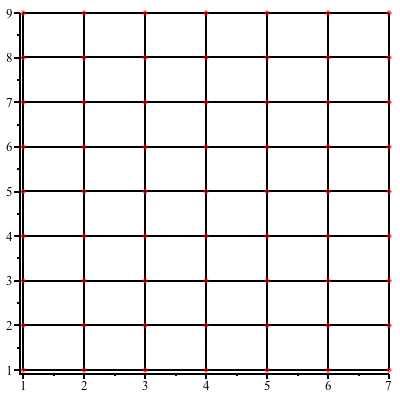The given data values corresponding to each data point are stored in data which is a 7x9 Array. A random collection of values is generated for this example.

 > data3D:=Array( LinearAlgebra:-RandomMatrix(7, 9, generator=0.2 .. 0.8), datatype=float):

The first 3-D plot below is the piecewise-planar surface produced by default. The goal is to produce a smoother surface instead, and this will be accomplished by using the gridsize and interpolation options of the surfdata command.

The plotted surface is being overlaid here with both the 3-D point-plot as well as the (linear) patch-surface, so that the computed surface may be visually demonstrated to be authentic with respect to the original discrete data.

 > ptsplot:=PLOT3D( GRID(1..7, 1..9, data3D, STYLE(POINT) ) ): gridplot:=PLOT3D( GRID( 1..7, 1..9, data3D, STYLE(WIREFRAME)) ):

The following plot3d options control the general look of these plots, and are reused in this subsection. They are assigned to a single, reusable name so that the differences between methods is more clearly illustrated.

 > lookandfeel := axes=boxed, style=surface, glossiness=1.0, lightmodel=light1,               color=RGB(204/255, 84/255, 0/255), view=[1..7, 1..9, 0..1]:

 > display(   ptsplot,gridplot,   surfdata(data3D, 1..7, 1..9, lookandfeel)                );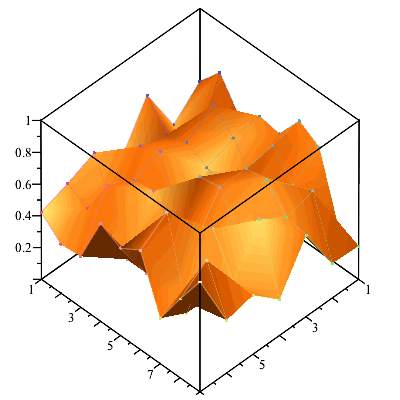On the left below is the default surface produced by the surfdata command, without any additional interpolation.

On the right below is the surface produced by the surfdata command using the new interpolating optional keyword parameters gridsize and interpolation.

display( Array([

surfdata( data3D, 1..7, 1..9, lookandfeel ),

surfdata( data3D, 1..7, 1..9,
gridsize=[28,36], interpolation=[method=spline],
lookandfeel)

]) );> display( Array([     display( ptsplot, gridplot,              surfdata( data3D, 1..7, 1..9, lookandfeel ) ),     display( ptsplot, gridplot,              surfdata( data3D, 1..7, 1..9,                        gridsize=[28,36], interpolation=[method=spline],                        lookandfeel) )                 ]) );Note that the 3-D plot renderer does its own small amount smoothing of the surface. Hence, even when using the purely linear method of the computational interpolation scheme, the plot on the right below shows a modest level of surface smoothing.

 > display( Array([     display( ptsplot, gridplot,              surfdata( data3D, 1..7, 1..9, lookandfeel ) ),     display( ptsplot, gridplot,              surfdata( data3D, 1..7, 1..9,                        gridsize=[28,36], interpolation=[method=linear],                        lookandfeel) )                 ]) );The refined grid on which values are interpolated does not need to contain a whole integer multiple (in either direction) of the number points of the original grid of data points.

 > display( Array([     display( ptsplot, gridplot,              surfdata( data3D, 1..7, 1..9, lookandfeel ) ),     display( ptsplot, gridplot,              surfdata( data3D, 1..7, 1..9,                        gridsize=[33,33], interpolation=[method=cubic],                        lookandfeel) )                 ]) );Non-uniform data grid

With new functionality in Maple 16, it is now possible to create an interpolate 3-D surface from irregularly spaced data.

Here below is an example. The data is supplied as an n-by-3 Array or Matrix. Each row represents a point, which the columns interpreted as x-, y-, and z-value. First, we'll look down upon the projection of the data points onto the x-y plane and thus visualize the layout and spacing of the 2-D dependent data values.

This is the same data that was used for the ScatterPlot3D example in the Smoothing section. But in contrast to the smoothing example we are now supposing that the data points are to be interpolated, which is to say that the surface must pass directly through the data points.

 > M:=ImportMatrix(cat(kernelopts(mapledir),"/data/plotting/irregulardata3D.csv"),                source=csv,datatype=float):

 > Ppt := pointplot3d(M,axes=box,style=patchnogrid,symbolsize=15,color=red,                          labels=[x,y,z]):

 > display(Ppt,orientation=[90,0,0]);The surfdata command can now handle this task directly.

The option `source=irregular` is supplied to denote that this data is not to be interpreted as forming a regularly spaced grid with only three values along one of the two independent data dimensions.

 > surfdata(M,source=irregular,axes=box);How to Proceed: Pricing & Purchase Evaluate Upgrade Get Price Quote Buy Online